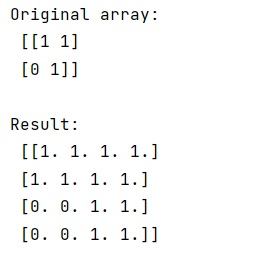# How to scale a NumPy array?

Learn, how can we scale a NumPy array?
Submitted by Pranit Sharma, on March 08, 2023

## Scaling a NumPy array

Suppose that we are given a numpy array of shape (n, m) and we need to scale this numpy array by a factor of k which results in an array of shapes (n*k, m*k).

That is, the value of each cell in the original array is copied into k corresponding cells in the resulting array. Assuming arbitrary array size and scaling factor, we need to find the most efficient way to do this.

To scale a NumPy array, we can use numpy.kron() which is used to compute the Kronecker product of two arrays which means to create a composite array made of blocks of the second array scaled by the first.

It takes two parameters a, b which are the input arrays for which the Kronecker product is computed.

We can create an array filled with ones of shape K by which we need to scale the original array and then we can apply numpy.kron() on both arrays.

Let us understand with the help of an example,

## Python code to scale a numpy array

```# Import numpy
import numpy as np

# Creating a numpy array
arr = np.array([[1, 1],[0, 1]])

# Display original array
print("Original array:\n",arr,"\n")

# Scaling up the original array
k = 2
res = np.kron(arr,np.ones((k,k)))

# Display result
print("Result:\n",res,"\n")
```

Output:Languages: » C » C++ » C++ STL » Java » Data Structure » C#.Net » Android » Kotlin » SQL
Web Technologies: » PHP » Python » JavaScript » CSS » Ajax » Node.js » Web programming/HTML
Solved programs: » C » C++ » DS » Java » C#
Aptitude que. & ans.: » C » C++ » Java » DBMS
Interview que. & ans.: » C » Embedded C » Java » SEO » HR
CS Subjects: » CS Basics » O.S. » Networks » DBMS » Embedded Systems » Cloud Computing
» Machine learning » CS Organizations » Linux » DOS
More: » Articles » Puzzles » News/Updates